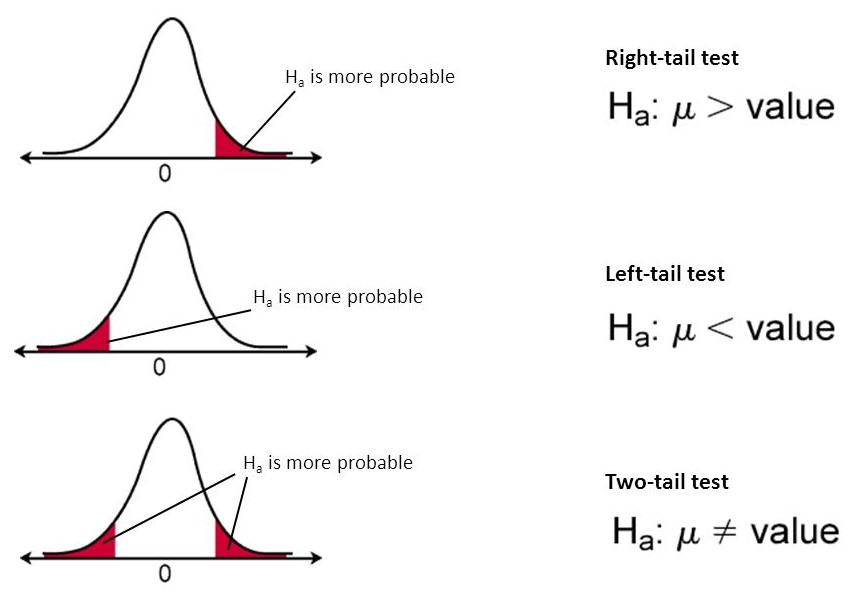Statistics

# Hypothesis Testing §

I learned this in Enriched bio a while ago at Marianopolis College, I regret not learning this more seriously, as I have forgotten all of it now. Update: I am learning about it in STAT206!

Null Hypothesis (): “Current belief”; conventional wisdom Alternate Hypothesis (): Challenge to

There are two types of test

• Two-tailed (what we stick to in STAT206, using )
• One-tailed (using inequality or )I finally understand this intuitively. If you fall within the region of rejection, then you reject your null hypothesis.

• We have enough evidence to support a claim that /

### 4-Step Method for Hypothesis Testing §

1. Construct the Test Statistic
2. Calculate the value of the Test Statistic
3. Compute the p-value
• This is a little hard and intimidating, I don’t know what values I should be looking at
• Okay, I am starting to get it. I think you need to be careful about which test to use. If you
• If you variance is known, use Z-Table.
• If your variance is unknown, use the T-table, where your DOF is .
• There is also DOF of if you are doing linear regression??
4. Draw appropriate conclusions for the -value
• reject , fail to reject
• We can also say “we have enough evidence to support
• fail to reject , reject
• You can’t say the other way around, since 0.05 is not enough. You need to have another test
• “There does not appear to be a difference between enough evidence to show that

Standard testing for a mean:

• There is not enough evidence to show a particular mean?? But that is kind of iffy

Comparing two means:

• Support of : There is not enough evidence to show that the means are the same
• Support of : There is not enough evidence to show that the means are different

Linear

• There seems to be linear relationships between X and Y.
• There is not enough evidence to show that a linear relationship exists between X and Y.

### Normal Hypothesis Testing §

Let’s illustrate the 4-step method through an example of normal hypothesis testing.

Suppose , s are independent. and . We want to test the following hypothesis:

Can we conclude that our sample data supports ?

##### Step 1: Construct the Test Statistic §

See Test Statistic for more information. We’ve seen this before in Test Statistic for more information. We’ve seen this before in Confidence Interval: You conclude that you have the following value:

##### Step 3: Compute the p-value §
• If you variance is known, use Z-Table.
• If your variance is unknown, use the T-table, where your DOF is .
P(Z \leq 2.1) &=0.98214\\ [3pt] \implies P(|Z| \geq 2.1)&=2(1-0.98214)\\ [3pt] \text{p-value}&=0.03572 \end{aligned}$$This is a little confusing, it's in the similar lens of the idea I talked about in [[notes/Confidence Interval|Confidence Interval]] when retrieving the right Z-value. ![[attachments/Screen Shot 2022-12-14 at 11.07.03 PM.png]] ##### Step 4: State your conclusion Since p < 0.05, we have strong strong evidence against H_0. Therefore, we reject H_0, and fail to reject H_1. If you want to test for unknown \sigma, you use the same idea as you saw in [[notes/Confidence Interval|Confidence Interval]], notes is page 347. You use the [[notes/Student's t-Distribution|t-table]]. ### Binomial Hypothesis Testing Ex: A survey of 1000 Americans asked who they would vote for; Biden (52%) or Trump (48%). Is this election too close to call? H_0: \theta = \frac{1}{2} H_1: \theta \neq \frac{1}{2}$$H \ Y = \# \ \text{of people voting for Biden} \implies Y \sim Bin(1000, \theta)$$Step 1: Construct the Test Statistic We use the [[notes/Pivotal Quantity|Pivotal Quantity]] for the binomial [[notes/Confidence Interval|Pivotal Quantity]] for the binomial [[notes/Confidence Interval|Confidence Interval]].$$\frac{Y - n\theta}{n\theta \sqrt{(1-\theta)}}= Z \sim N(0,1)\implies Z = \frac{Y - 1000 \cdot \frac{1}{2}}{\sqrt{1000 \cdot \frac{1}{2}\cdot \frac{1}{2}}}\implies D = \left| \frac{Y-500}{\sqrt{250}} \right |$$Step 2: Calculate the [[notes/Test Statistic|Test Statistic]]$$d = \left| \frac{520-500}{\sqrt{250}}\right| \approx 1.26 ### Relationship with [[notes/Confidence Interval|Confidence Interval]] So they are the same idea? 1. A 95% [[notes/Confidence Interval|Confidence Interval]] 2. Conducting a [[notes/Hypothesis Testing|Hypothesis Test]] using a 0.05 cutoff for the [[notes/p-value|p-value]] - $H_0: \theta = \theta_0$ - $H_1: \theta \neq \theta_0$ - For the CI, we find $\theta_0 \pm a$ - For hypothesis test, if $\theta$ is in $\theta_0 \pm a$, then we conclude $H_0$. Else, we conclude $H_1$. ### Hypothesis Testing with Two Means This is where I am getting confused. ### Related - [[notes/Test Statistic|Test Statistic]] - [[notes/Type I and Type II Error|Type I and Type II Error]] - [[notes/Chi-Squared Distribution|Chi-Squared Distribution]] - Chi-Square Goodness of fit test - [[notes/p-value|p-value]] - [[notes/Z-Score|Z-Score]]